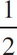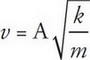# AP Physics 1 Question 85: Answer and Explanation

### Test Information

Question: 85

5. In a spring-block oscillator, the maximum speed of the block is

• A. proportional to amplitude
• B. proportional to the square of amplitude
• C. proportional to the square root of amplitude
• D. inversely proportional to the square root of amplitudemv2max =kA2